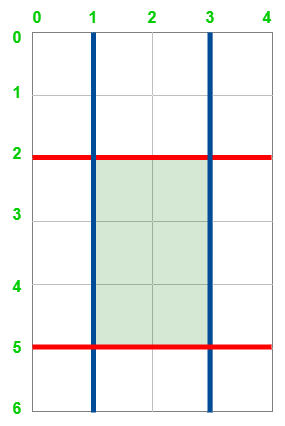Open In App

# Maximum area of a Cake after Horizontal and Vertical cuts

Given two positive integers h and w representing the height h and width w which forms a rectangle. Also, there are two arrays of integers horizontalCuts and verticalCuts where horizontalCuts[i] is the distance from the top of the rectangle to the ith horizontal cut and similarly, verticalCuts[j] is the distance from the left of the rectangle to the jth vertical cut. The task is to find the maximum area of the rectangle after you cut at each horizontal and vertical position provided in the arrays horizontalCuts and verticalCuts. Since the answer can be a huge number, return this modulo 10^9 + 7.

Examples :max area = 6

Input: h = 6, w = 4, horizontalCuts = [2, 5], verticalCuts = [1, 3]
Output: 6
Explanation: The figure above represents the given rectangle. Red lines are the horizontal cuts and blue lines are vertical cuts. After the rectangle is cut, the green piece of rectangle has the maximum area.

Input: h = 5, w = 4, horizontalCuts = [3, 1], verticalCuts = 
Output: 9

Approach: The problem can be solved by observing that-

• The horizontalCuts if perpendicular to any VerticalCut, then all the vertical slices cross all the horizontalCuts.
• Next, the maximum area of the rectangle must be enclosed by at least one vertical and one horizontal cut.

From the above observation, it is clear that we need to find the maximum distance between two horizontal cuts and two vertical cuts respectively, and multiply them to find the area of the rectangle. Follow the steps below to solve the problem:

• Sort both horizontalCuts and verticalCuts lists.
• Initialize two variables, say MaxHorizontal and MaxVertical as horizontalCuts and verticalCuts respectively, as to consider the closest rectangles towards axis both horizontally and vertically which will store the maximum horizontal and vertical lengths of the rectangle respectively.
• Iterate in the range [1, horizontalCuts.size()-1] using the variable i and perform the following steps:
• Modify the value of MaxHorizontal as max(MaxHorizontal, horizontalCuts[i] – horizontalCuts[i-1]).
• Modify the value of MaxVertical as max(MaxVertical, verticalCuts[i] – verticalCuts[i-1]).
• Print MaxHorizontal*MaxVertical as the answer.

Below is the implementation of the above approach:

## C++

 `// C++ Program for the above approach``#include ``using` `namespace` `std;``const` `int` `mod = 1e9 + 7;` `class` `Solution {``public``:``    ``// Returns the maximum area of rectangle``    ``// after Horizontal and Vertical Cuts``    ``int` `maxArea(``int` `h, ``int` `w, vector<``int``>& horizontalCuts,``                ``vector<``int``>& verticalCuts)``    ``{` `        ``// Sort the two arrays``        ``sort(horizontalCuts.begin(), horizontalCuts.end());``        ``sort(verticalCuts.begin(), verticalCuts.end());` `        ``// Insert the right bound h and w``        ``// in their respective vectors``        ``horizontalCuts.push_back(h);``        ``verticalCuts.push_back(w);``        ` `          ``//Initialising both by first indexs,``          ``//to consider first rectangle formed by``          ``//respective horizontal and vertical cuts``        ``int` `maxHorizontal = horizontalCuts;``        ``int` `maxVertical = verticalCuts;` `        ``// Find the maximum Horizontal Length possible``        ``for` `(``int` `i = 1; i < horizontalCuts.size(); i++) {``            ``int` `diff``                ``= horizontalCuts[i] - horizontalCuts[i - 1];``            ``maxHorizontal = max(maxHorizontal, diff);``        ``}` `        ``// Find the maximum vertical Length possible``        ``for` `(``int` `i = 1; i < verticalCuts.size(); i++) {``            ``int` `diff``                ``= verticalCuts[i] - verticalCuts[i - 1];``            ``maxVertical = max(maxVertical, diff);``        ``}` `        ``// Return the maximum area of rectangle``        ``return` `(``int``)((``long``)maxHorizontal * maxVertical``                     ``% mod);``    ``}``};` `// Driver Code``int` `main()``{``    ``// Class Call``    ``Solution ob;``  ` `    ``// Given Input``    ``vector<``int``> hc = { 2, 5 }, vc = { 1, 3 };``    ``int` `h = 6, v = 4;``    ``// Function Call``    ``cout << (ob.maxArea(6, 4, hc, vc));``    ``return` `0;``}`

## Java

 `// Java program for above approach``import` `java.util.*;``class` `GFG{``    ``// Returns the maximum area of rectangle``    ``// after Horizontal and Vertical Cuts``    ``public` `static` `int` `maxArea(``int` `h, ``int` `w, ArrayList horizontalCuts,``                    ``ArrayList verticalCuts)``    ``{``        ``// Sort the two arrays``        ``Collections.sort(horizontalCuts);``        ``Collections.sort(verticalCuts);` `        ``// Insert the right bound h and w``        ``// in their respective vectors``          ` `          ``//if the set is empty, add 0 as default value``          ``if``(horizontalCuts.size() == ``0``){``            ``horizontalCuts.add(``0``);``          ``}``        ``if``(verticalCuts.size() == ``0``){``              ``verticalCuts.add(``0``);``        ``}``        ``horizontalCuts.add(h);``        ``verticalCuts.add(w);` `        ``// int maxHorizontal = 0;``        ``// int maxVertical = 0;``           ` `        ``int` `maxHorizontal = horizontalCuts.get(``0``);``        ``int` `maxVertical = verticalCuts.get(``0``);` `        ``// Find the maximum Horizontal Length possible``        ``for` `(``int` `i = ``1``; i < horizontalCuts.size(); i++) {``            ``int` `diff``                    ``= horizontalCuts.get(i) - horizontalCuts.get(i-``1``);``            ``maxHorizontal = Math.max(maxHorizontal, diff);``        ``}` `        ``// Find the maximum vertical Length possible``        ``for` `(``int` `i = ``1``; i < verticalCuts.size(); i++) {``            ``int` `diff``                    ``= verticalCuts.get(i) - verticalCuts.get(i - ``1``);``            ``maxVertical = Math.max(maxVertical, diff);``        ``}` `        ``// Return the maximum area of rectangle``        ``return` `(``int``)((``long``)maxHorizontal * maxVertical);``    ``}``    ``// Driver Code``    ``public` `static` `void` `main(String[] args)``    ``{``        ``// Given Input``        ``ArrayList hc = ``new` `ArrayList<>();``        ``hc.add(``3``);``        ` `        ``ArrayList vc = ``new` `ArrayList<>();``        ``vc.add(``3``);``        ``int` `h = ``5``, v = ``4``;``      ` `        ``// Function Call``        ``System.out.println(maxArea(``6``, ``4``, hc, vc));``    ``}``}` `//This code is contributed by Piyush Anand.`

## Python3

 `# python 3 Program for the above approach``mod ``=` `1000000007` `# Returns the maximum area of rectangle``# after Horizontal and Vertical Cuts``def` `maxArea(h, w, horizontalCuts,``            ``verticalCuts):` `    ``# Sort the two arrays``    ``horizontalCuts.sort()``    ``verticalCuts.sort()` `    ``# Insert the right bound h and w``    ``# in their respective vectors``    ``horizontalCuts.append(h)``    ``verticalCuts.append(w)` `    ``maxHorizontal ``=` `0``    ``maxVertical ``=` `0` `    ``# Find the maximum Horizontal Length possible``    ``for` `i ``in` `range``(``1``, ``len``(horizontalCuts)):` `        ``diff ``=` `horizontalCuts[i] ``-` `horizontalCuts[i ``-` `1``]``        ``maxHorizontal ``=` `max``(maxHorizontal, diff)` `    ``# Find the maximum vertical Length possible``    ``for` `i ``in` `range``(``1``,``                   ``len``(verticalCuts)):``        ``diff ``=` `verticalCuts[i] ``-` `verticalCuts[i ``-` `1``]``        ``maxVertical ``=` `max``(maxVertical, diff)` `    ``# Return the maximum area of rectangle``    ``return` `(``int``)(maxHorizontal ``*` `maxVertical``                 ``%` `mod)`  `# Driver Code``if` `__name__ ``=``=` `"__main__"``:` `    ``# Given Input``    ``hc ``=` `[``2``, ``5``]``    ``vc ``=` `[``1``, ``3``]``    ``h ``=` `6``    ``v ``=` `4``    ` `    ``# Function Call``    ``print``(maxArea(``6``, ``4``, hc, vc))` `    ``# This code is contributed by ukasp.`

## C#

 `// C# Program for the above approach``using` `System;``using` `System.Collections;``using` `System.Collections.Generic;``class` `GFG``{``    ``static` `int` `mod = 1000000007;``    ` `    ``// Returns the maximum area of rectangle``    ``// after Horizontal and Vertical Cuts``    ``static` `int` `maxArea(``int` `h, ``int` `w, List<``int``> horizontalCuts, List<``int``> verticalCuts)``    ``{``        ``// Sort the two arrays``        ``horizontalCuts.Sort();``        ``verticalCuts.Sort();``     ` `        ``// Insert the right bound h and w``        ``// in their respective vectors``        ``horizontalCuts.Add(h);``        ``verticalCuts.Add(w);``     ` `        ``int` `maxHorizontal = 0;``        ``int` `maxVertical = 0;``     ` `        ``// Find the maximum Horizontal Length possible``        ``for``(``int` `i = 1; i < horizontalCuts.Count; i++)``        ``{``     ` `            ``int` `diff = horizontalCuts[i] - horizontalCuts[i - 1];``            ``maxHorizontal = Math.Max(maxHorizontal, diff);``        ``}``     ` `        ``// Find the maximum vertical Length possible``        ``for``(``int` `i = 1; i < verticalCuts.Count; i++)``        ``{``            ``int` `diff = verticalCuts[i] - verticalCuts[i - 1];``            ``maxVertical = Math.Max(maxVertical, diff);``        ``}``     ` `        ``// Return the maximum area of rectangle``        ``return` `(``int``)(maxHorizontal * maxVertical % mod);``    ``}``    ` `  ``static` `void` `Main ()``  ``{``    ``// Given Input``    ``List<``int``> hc = ``new` `List<``int``>(``new` `int``[]{ 2, 5 });``    ``List<``int``> vc = ``new` `List<``int``>(``new` `int``[]{ 1, 3 });``    ` `    ``// Function Call``    ``Console.WriteLine(maxArea(6, 4, hc, vc));``  ``}``}` `// This code is contributed by suresh07.`

## Javascript

 ``

Output

`6`

Time Complexity: O(NlogN)
Auxiliary Space: O(1)